Browse by Topic
Related Topics

# In Defense of Infinity

The Glencoe Algebra II study materials (p. 10) make an amazing claim (Reddit).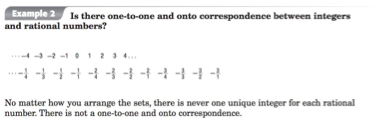This statement is in a math textbook, but it is horrifyingly wrong. A statement like “the letters A–Z cannot be matched up with the numbers 1–26” would be similarly wrong. These two sets of the same size (here, 26) can be matched up as A1, B2, C3, …, Z26. Can the rational numbers be matched up with the integers? Both are infinite, which allows for the tricks of a technique called Hilbert’s hotel, a hotel with infinite numbered rooms that can always make room for one more guest. The Glencoe claim asks if the cardinality of the integers and rationals is the same. Both are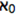, or Aleph-0, which Georg Cantor proved in the 1870s.

A “one-to-one and onto” correspondence means that each rational number is paired with exactly one integer and every integer is accounted for. Find such a correspondence, and you’ve shown that each set contains the same number of elements.

Since there are negative numbers in the Glencoe worksheet, let’s pair negative integers to negative rationals and positive integers to positive rationals. One method for pairing positive rational numbers with positive integers is to arrange the rationals on a grid and then to make a zigzag triangle, or in other words, by enumerating the rational numbers (code below by John Stucky).

This technique uses a pairing function to find a path through the lattice points in a quadrant. It is one of the functions that enumerate pairs. In this enumeration, the fraction 5/6 pairs with the integer 37.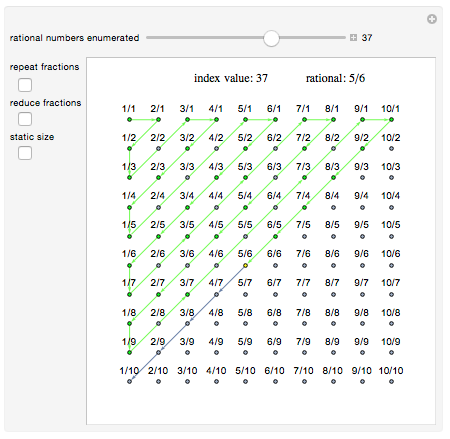There are more ways of showing that the integers and rationals have the same cardinality.

The Calkin–Wilf tree of fractions pairs binary representations of the integers with rational numbers. Each fractionhas 0 and 1 branches, namely,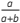and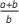. The binary numbers start out 1, 10, 11, 100, 101, …—corresponding to the integers 1, 2, 3, 4, 5, …—which give paths through the tree to the rational number they are paired with. With the base of the Calkin–Wilf tree being 1, the binary number 101, for example, specifies the path starting at the base (1), going left (0), and then right (1) to arrive at 3/2. Counting in binary gives the sequence of rationals 1, 1/2, 2, 1/3, 3/2, 2/3, 3. Every rational number occurs in the tree.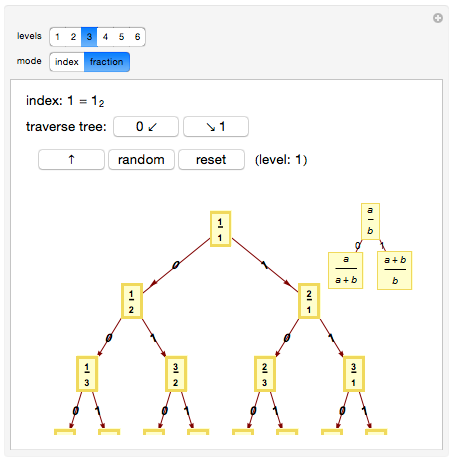Another method for enumerating the rationals was found independently by German mathematician Moritz Stern in 1858 and French clockmaker Achille Brocot in 1860, and is now known as the Stern–Brocot tree (code by Michael Schreiber). Take a look at 5/8. There are spaces to either side of 5/8. Above those spaces are the fractions 2/3 and 3/5. The rational 5/8 = (2+3)/(3+5). This way of combining fractions is called “child’s addition” or the “mediant.” The invisible fractions 0/1 and 1/0 are considered to be the far left and far right spaces. With child’s addition, 0/1+1/4 = 1/5, and 4/1+1/0 = 5/1.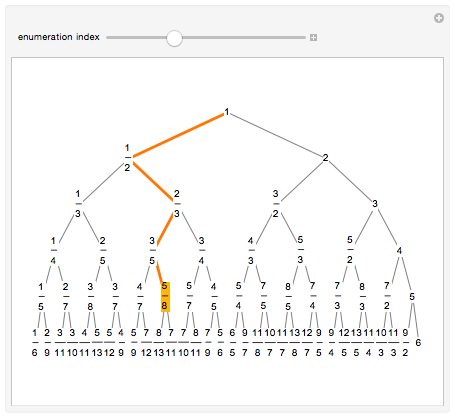The Manipulate below covers code for converting any reduced rational number into a unique integer, and for converting any integer into a unique rational number. What is the “other system?” Let Calkin–Wilf and Stern–Brocot be functions CW(x) and SB(x), turning rationals to integers and integers to rationals. It turns out that CW(SB(x)) = SB(CW(x)), and this value is listed as “other system.”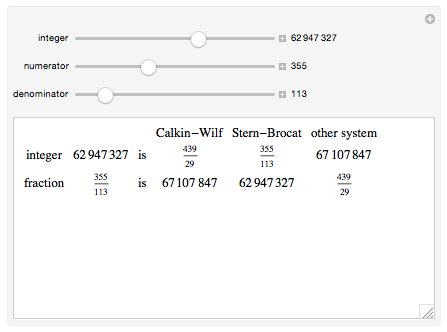With the code available in the downloadable CDF at the end of the post, integers can be turned into rationals.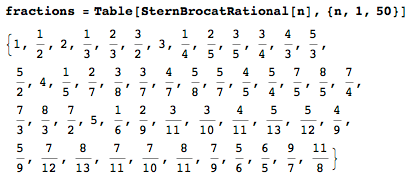Rationals can be turned into integers. The “one-to-one and onto” relations are thus strongly demonstrated.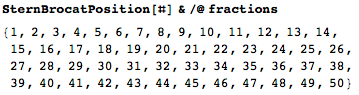In addition to the zigzag triangle, Stern–Brocot, and Calkin–Wilf, there is also the Bird tree. It’s possible that the Glencoe Algebra II authors were thinking of the real numbers, which can be proven uncountable by Cantor’s diagonalization method. In any event, these methods for enumerating the rational numbers are well known. The authors and editors of Glencoe Algebra II should have been aware of them.

As long as we’ve got the code out, let’s have some fun with it. Plots of the Calkin–Wilf fractions look like the marks on a ruler.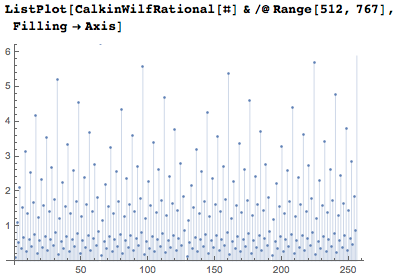Plots of the Stern–Brocot fractions have sections that are related to Minkowski’s question mark function and the Devil’s staircase.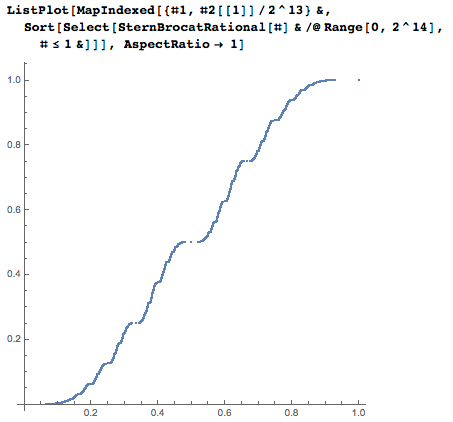With a scatterplot, the two sequences together make an interesting pattern.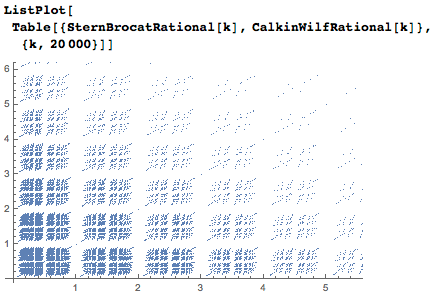If x is a fraction in the Calkin–Wilf sequence, the next fraction is 1/(2 ⌊x⌋ – x+ 1), with ⌊x⌋ being the floor function (Gosper). The previous fraction is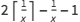. Also, notice that the denominator of one term is the numerator of the next term.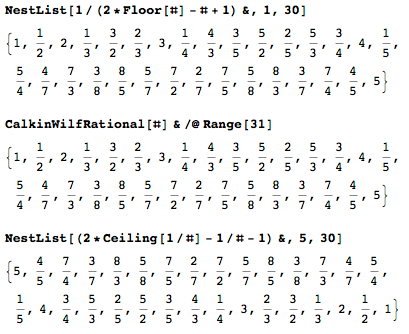As an experiment, find the integer corresponding to a rational number, convert that number into binary digits, reverse the digits, split them into groups of similar numbers, and then find the length of each group.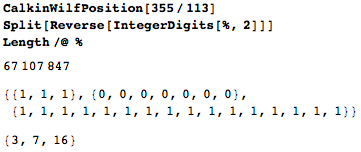As another experiment, find the continued fraction of the same rational.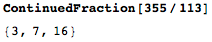See the relation?